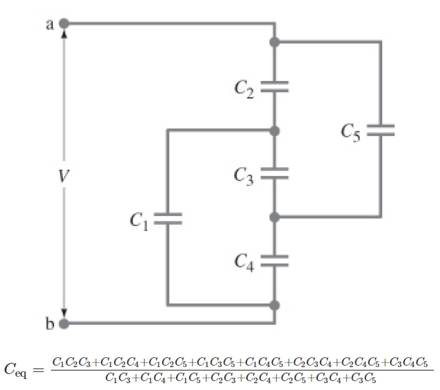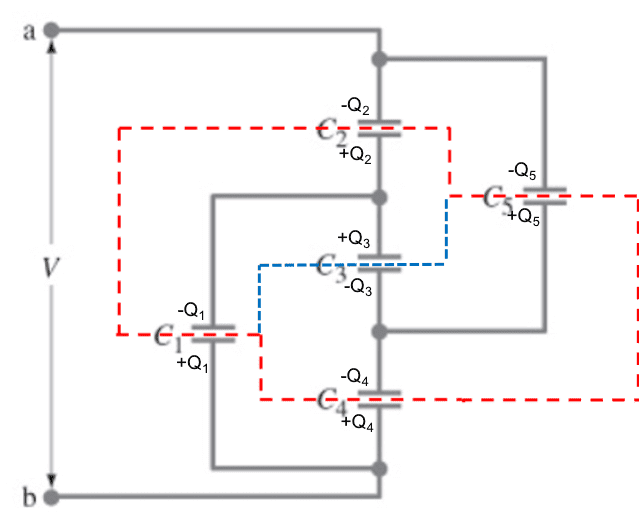# Finding the Total Capacitance

Homework Statement:
Show that total capacitance is equal to C_eq below.
Relevant Equations:
C_eq= C_1+C_2+C_3+...+C_n (parallel capacitors)

C_eq^(-1)= C_1^(-1)+C_2^(-1)+...+C_n^(-1) (serial capacitors)## Answers and Replies

BvU
Science Advisor
Homework Helper
Hello @JJJAKE ,!

Physics forum is not an agency that does your homework for you. You'll have to make an effort yourself first.

So: what can you do to make an inroad to this exercise ? Perhaps redraw the diagram a little ?

(as a welcome present:) Your relevant equations are not. You'll need something more elementary.

Last edited:
I can't understand which ones are parallel or which ones are series. I can say Qtotal=Q1+Q4=Q2+Q5 but not sure about Q3 because the current way is unknown at C3. Because of that, I can't calculate from V values. This is not my homework by the way, It is a practice question from Pearson's physic book.

BvU
Science Advisor
Homework Helper
The relevant equation in this case is Q = CV.
What about my suggestion to re-draw the diagram ? How would it look if one particularC wasn't there ?

(bit cryptic, I concede, but when I give it away the fun is gone, for you too)

lewando
Homework Helper
Gold Member
I'm afraid there is no symmetry here. This is the general (worst) case. A capacitor cannot disappear if there is no symmetry. Agree with BvU that redrawing this would be helpful, in that it would reveal an arrangement that would inspire the use of another relevant equation useful in cases where nothing is in series nor in parallel.

kuruman
Science Advisor
Homework Helper
Gold Member
An alternate method to the general (worst) case would be to use the Kirchhoff voltage law (KVL) around closed loops and replace the current conservation at nodes with charge conservation. When the voltage source is connected, parts of the circuit that were neutral and electrically isolated remain neutral overall as charges are induced within them to and from capacitor plates. So if one constructs appropriate Gaussian surfaces, one can demand that the sum of enclosed charges be zero. In OP's modified figure below, it is assumed that ##V_b>V_a##. This assumption sets the polarity of all plates except ##C_3##. When specific numbers are substituted, the sign of ##Q_3## will indicate whether the guess was good or bad.Sample equations:
##Q_2-Q_1-Q_4+Q_5=0## (red Gaussian outline)
##V-\dfrac{Q_1}{C_1}-\dfrac{Q_2}{C_2}=0## (KVL for leftmost loop)
As usual, one should watch out for redundancy. Here, only two of the three Gaussian loops are usable. It is also clear from the drawing that ##Q_1+Q_4=Q_2+Q_5=Q_{tot}##. This reduces the number of equations and unknowns.

I believe no one here knows how to solve the problem.

•BvU
berkeman
Mentor
I believe no one here knows how to solve the problem.
When tricks don't work, what is left? Show us your KCL equations for an AC input source please...

•BvU
berkeman
Mentor
After a thread cleanup, the OP has left the building and the thread will be closed now. Thanks folks for trying to help the poster.